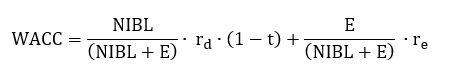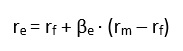## Review questions

Cost of capital is useful in many contexts:

• Valuation
• Credit analysis
• Remuneration
• Hurdle rate for investment projects
• Benchmark in performance measurement
• Etc.

The capital structure refers to the proportion of net interest bearing liabilities (NIBL) to equity. The capital structure has an impact on shareholders return and the cost of capital. For instance, cost of equity and the capital structure defined as NIBL to equity are positively correlated.

Beta refers to the systematic risk of a firm; i.e. the risk that cannot be diversified away through the holding of a portfolio of assets. It is only the systematic risk which is priced. A beta equal to one reflects that the asset has a risk similar to the market (average). A beta above one (for instance a beta of 2.0) suggests that the risk is above the average risk of the market.

There are broadly two fundamental risk factors which should be considered:

• Operating risk
• Financial risk

Operating risk is affected by three risk factors – external risk, strategic risk and operational risk

Financial risk is affected by (broadly) two factors – financial leverage and loan characteristics (for instance variable versus fixed interest rate, maturity and currency)

WACC is defined asWhere

NIBL = (Market value of) net interest bearing liabilities (net financing items)

E = (Market value of) equity

rd = Required rate of return on net interest bearing liabilities

re = Required rate of return on equity

t = Corporate tax rate

Most financial textbooks suggest using the Capital Asset Pricing Model (CAPM) when estimating the investors’ required rate of return. According to CAPM investors’ required rate of return is defined as:where

re = Investors’ required rate of return

rf = Risk-free interest rate

βe = Systematic risk on equity (levered beta)

rm = Return on the market portfolio

Theoretically, the best estimate of the risk free rate would be the expected return on a zero-beta portfolio. In reality, most analysts apply a single yield to maturity from a government bond that best matches the cash flows being valued or analysed. Although there are different government bonds to choose from, zero-coupon government bond are generally preferred. They are also known as stripped bonds or strips. This is motivated by the fact that the maturity is better established than alternative bonds and reinvestment risk is avoided. For valuation purposes, where the time horizon is often infinite, the risk free rate is proxied by 10-year or 30 year government zero-coupon government bond. While the 30 year government bond often matches the underlying cash flow better, it may also suffer from illiquidity which affects the yields. To handle issues such as inflation it is important that the government bond is denominated in the same currency as the underlying cash flows. This implies that local government bonds should be applied.

There are typically two ways in which the risk premium can be determined:

• Ex-post approach
• Ex-ante approach